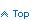See if ADO.NET DataSet contents changed using the GetChanges method

You have a DataGrid bound to a DataSet that allows users to add, delete and modify rows. When the form closes you want to know if data was changed so you can prompt the user to save their data (assuming you post back all database changes yourself which is what True programmers do).

You can check for changes using the following code which returns TRUE if any data changed.

```    Private Function DataSetChanged(ByVal theDataset As DataSet) As Boolean
Dim dtChanges As DataTable
Dim blnDataChanged As Boolean = False
'
' Create a table containing the changed rows.
'
dtChanges = theDataset.Tables(0).GetChanges
'
' See if the table contains changed rows.
'
If dtChanges Is Nothing Then
Return False
Else
'
' If rows were added or deleted we have confirmed changes.
' If a value changed confirm it changed. "RowState" will indicate
' a change even if the value was reset to its original value
'
Dim Row As DataRow
Dim intColumn As Integer

For Each Row In dtChanges.Rows
Select Case Row.RowState
blnDataChanged = True

Case DataRowState.Deleted
blnDataChanged = True

Case DataRowState.Modified
For intColumn = 0 To dtChanges.Columns.Count - 1
If Row(intColumn, DataRowVersion.Original) <> _
Row(intColumn, DataRowVersion.Current) Then

blnDataChanged = True
Exit For
End If
Next
End Select
If blnDataChanged Then Exit For
Next
Return blnDataChanged
End If
End Function
```